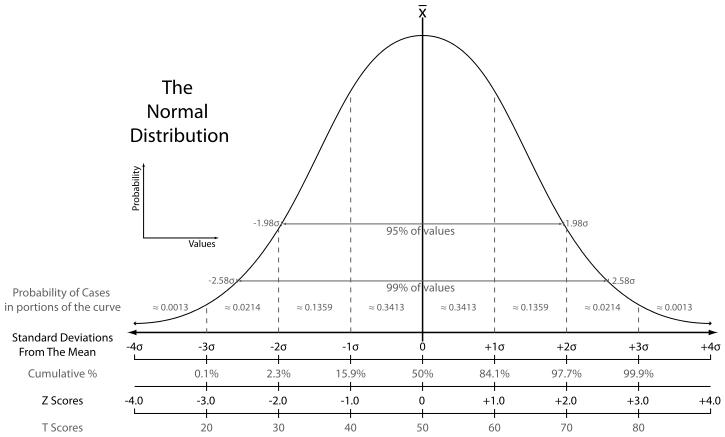# Data Distributions in R

#### Basic SyntaxData distributions in R provide many functions to generate and test random samples.  For any distribution, it is possible to use the density, cumulative probability, quartiles, or a random number generator.  The distribution name must be prefaced with the letters d, p, q or r as follows:

• Density: d<distrib.name>()
• Cumulative Probability: p<distrib.name>()
• Quartile: q<distrib.name>()
• Random Number: r<distrib.name>()

#### Data Distributions in R

A list of data distributions in R appears below.  Additional distributions are found in many packages listed on CRAN:

NameDescriptionParametersDefaults
beta()Betashape1, shape2-, -
binom()Binomialsize, prob-, -
Cauchy()Cauchylocation, scale0, 1
chisq()ChiSquareDf-
exp()Exponentialrate 1
f()Fdf1, df2-, -
gamma()GammaShape-
geom()GeometricProb-
hyper()Hypergeometricm, n, k-, -, -
lnorm()Lognormalmean, sd (of log)0, 1
multinomMultinomialn variables, size-, -
nbinomNegative binomialsize, prob-, -
norm()Normalmean, sd0, 1
pois()PoissonLambda-
T()Student TDf-
unif()Uniformmin, max0, 1
weibull()WeibullShape-
Wilcox()Wilcoxm, n-, -

#### Repeating Random Draws

The .Random.seed object is reset after each call to a random number function.  To reproduce the same random number sequence, the .Random.seed object must be assigned and saved for re-use.  Alternatively, the user must “fix” the set.seed() function with an integer.  The examples below will clarify:

The alternative approach to repeating random sequences uses the .Random.seed object as follows: